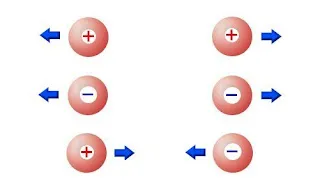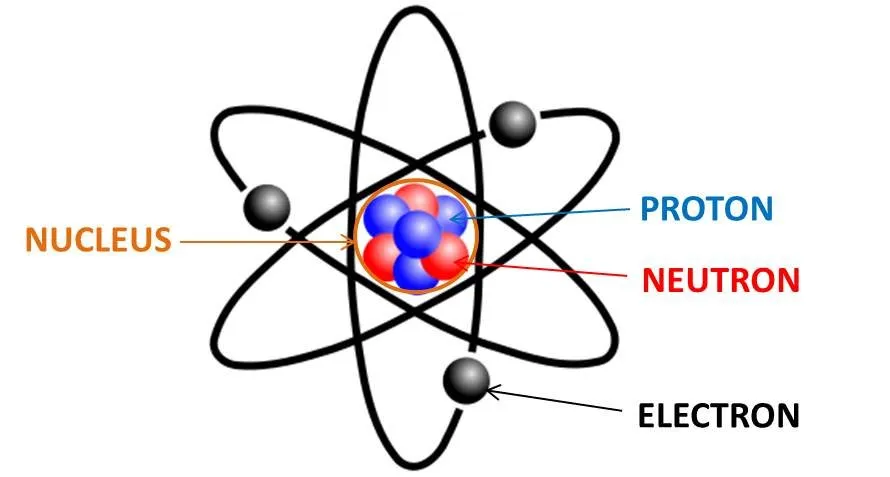# Physics - Electromagnetism - Getting into ElectromagnetismSource

## Introduction

Hello it's a me again Drifter Programming!
This time we continue with my Physics "category" and more specifically we get into a new branch! Previously in Physics posts I covered Classical Mechanics which where about motion and Newton's motion laws. There is a lot more to talk about this "previous" branch too, but just for some more diversity I'm thinking of starting out with some Electromagnetism too!

Today's post will be a small introduction to this new branch where we will get into what Electromagnetism studies and some basic theory about electric charge, conductors, insulators and also electric quantization.

So, without further do, let's get started!

## What does Electromagnetism study?

Electromagnetism is a physics branch that studies electromagnetic forces and so the physical interaction between electrically charged particles

From wikipedia

The electromagnetic force is part of the weak nuclear force when talking about fundamental forces.

So, to get more specific Electromagnetism studies:

• Electric charges, fields and forces
• Electric flux (Gauss's law)
• Electric potential energy and potential
• Capacitance and Dielectrics
• Electric current, resistance and electromotive force
• Direct current circuits (Kirchhoff's laws)
• Magnetic fields and forces
• Electromagnetic field sources
• Electromagnetic induction
• Self-Inductance
• Alternating current
• Electromagnetic waves

This is all that I will cover about Electromagnetism.

You can see that there is a looot to talk about and so this branch will take up a lot of time.

So, after this small introduction let's now get into some theory.

## Electric chargeSource

The first thing that you should ask yourself is what electric charges are.

The Ancient greeks saw that by rubbing amber with hair the amber started to attract/pull objects. Today we say that the amber has been charged or that it acquired electric charge.

During even more of those experiments (like rubbing plastic with cloth) scientists got into the conclusion that:

Two positive or two negative charged objects repel each other. One positive and one negative attract each other.

Benjamin Franklin

To explain that previous bit a little better we also have to explain what happens during those experiments. When rubbing two objects, electric charge moves from one object to the other and so one of the objects gets positively charged and the other negatively charged. (actually electrons move from one to the other and so one has "more" electrons and the other "less" electrons, but this doesn't mean that protons can't move!)

For example when rubbing plastic with coat both objects are being charged and also have the same meter of charge, but will have an "opposite" sign of charge

So, this means that we don't create charge, but we just move it.

This last bit is also a part of the electric charge conservation that we will get back to, by the end of this post.

## Conductors, Insulators, Semiconductors

Some materials let this electric charge move through them, whilst others don't.

That way we end up with the following categories:

• Materials that let this electric charge pass through are called Conductors.
• Materials that don't let electric charge pass through them are called Insulators
• Materials that are neither Conductors nor Insulators, but are something in between depending on the situtation and are called Semiconductors

Conductors are most metals, whereas Insulators are most non-metal materials.

A commonly known Semiconductor is silicon that is used in all the chips (transistors) that we have today!

So, this categorization now makes us think of the previous experiments again in another way.

For example when rubbing amber (metal) with our hair (non-metal) electric charge moves from the hair to the amber, causing the amber to get negatively charged, whilst the hair gets positively charged.

The electric charge that moves is the so called inductive charge and this whole procedure is called charge by Induction and we will get back to it posts later on where we will get into Self-Induction...

## Electric QuantizationSource

One final thing that needs to be covered is the conservation of electric charge.

Talking about all this stuff previously we showed how this electric charge moves, but we don't talked about what actually moves.

Those electric interactions are responsible of the structure of atoms and molecules and so are one of the fundamental characteristics of the particles from which all matter is build up.

Common matter is build up of electrons, protons and neutrons. Those are build up of quarks etc. that we will cover more specifically in my Atomic and Molecular Physics. Either way protons and neutrons build up the nucleus (core) and electrons travel around it. Protons are positively charged, electrons are negatively charged and neutrons are neutral.

The number of protons or electrons of the neutrally charged atom is called the atomic number.

Electric charge is actually the electrons moving from one atom to another and so when an atom gets electrons (it's easier to lose them, cause they are moving) it gets negatively charged and becomes an negative ion. The same way when it loses electrons it gest positively charged and becomes an positive ion.

In nature being charged is unstable and so most matter is neutrally charged and so not an ion. This means that the algebraic sum of all the electric charge of any closed system (for example an atom/material) is constant.

This exactly describes the conservation of electric charge.

So,

Electric charge can be moved from one atom to another, but it cannot be created or destroyed.

By now this principle has not been violated and so even with anti-matter (atoms build up of anti-electrons/positrons and anti-protons).

Lastly we can also say that the whole electric charge is quantized (electric quantization).

Only specific particles (electrons or positrons) are moving to cause electric charge and so any electric charge is the multiply of the charge caused by one electron (or positron).

Think of it like money, we can have 10 cents, but we can't have 11.5 cents when talking about 1 cent building up all of our money (well when getting into cryptocurrencies some cost less then 1 cent, but you get the idea).

Intro

Physics Introduction -> what is physics?, Models, Measuring

Vector Math and Operations -> Vector mathematics and operations (actually mathematical analysis, but I don't got into that before-hand :P)

Classical Mechanics

Velocity and acceleration in a rectlinear motion -> velocity, accelaration and averages of those

Rectlinear motion with constant accelaration and free falling -> const accelaration motion and free fall

Rectlinear motion with variable acceleration and velocity relativity -> integrations to calculate pos and velocity, relative velocity

Rectlinear motion exercises -> examples and tasks in rectlinear motion

Position, velocity and acceleration vectors in a plane motion -> position, velocity and accelaration in plane motion

Projectile motion as a plane motion -> missile/bullet motion as a plane motion

Smooth Circular motion -> smooth circular motion theory

Plane motion exercises -> examples and tasks in plane motions

Force and Newton's first law -> force, 1st law

Mass and Newton's second law -> mass, 2nd law

Newton's 3rd law and mass vs weight -> mass vs weight, 3rd law, friction

Applying Newton's Laws -> free-body diagram, point equilibrium and 2nd law applications

Contact forces and friction -> contact force, friction

Dynamics of Circular motion -> circular motion dynamics, applications

Object equilibrium and 2nd law application examples -> examples of object equilibrium and 2nd law applications

Contact force and friction examples -> exercises in force and friction

Circular dynamic and vertical circle motion examples -> exercises in circular dynamics

Electromagnetism

This is the first post of this branch :)

And this is actually it for today and I hope that you enjoyed it!

Next time we will cover Coulomb's law in depth with examples!

Bye!

H2
H3
H4
3 columns
2 columns
1 column